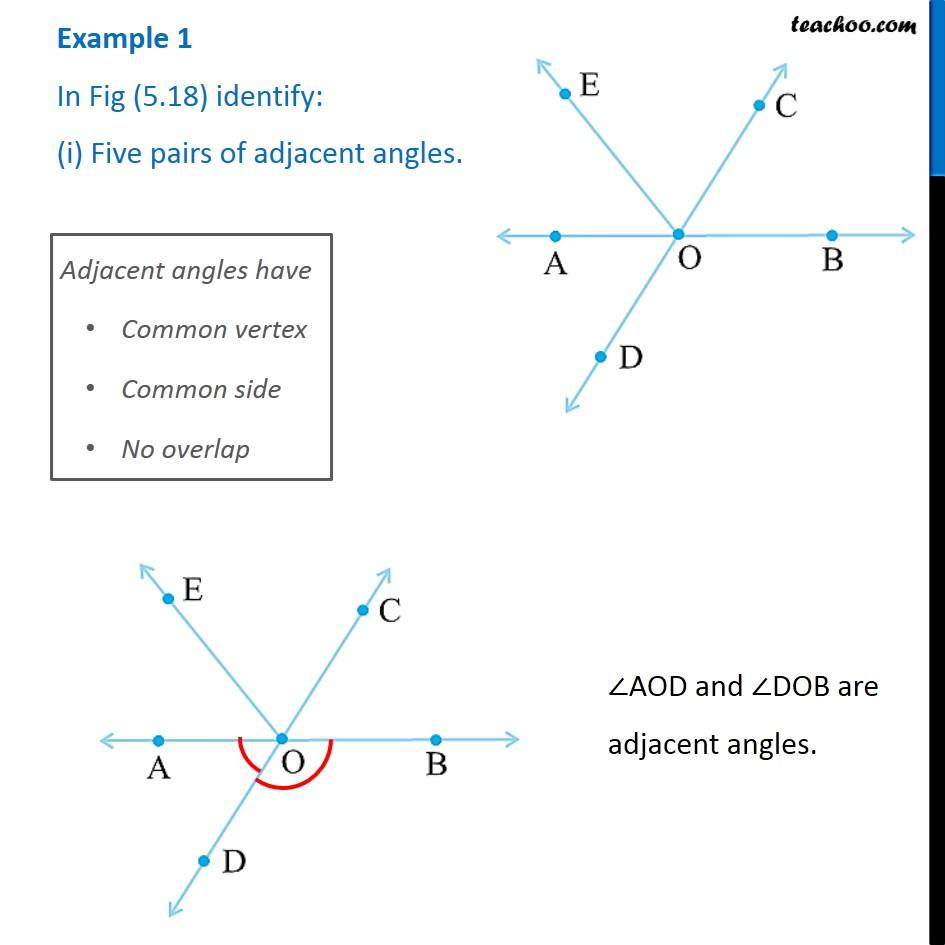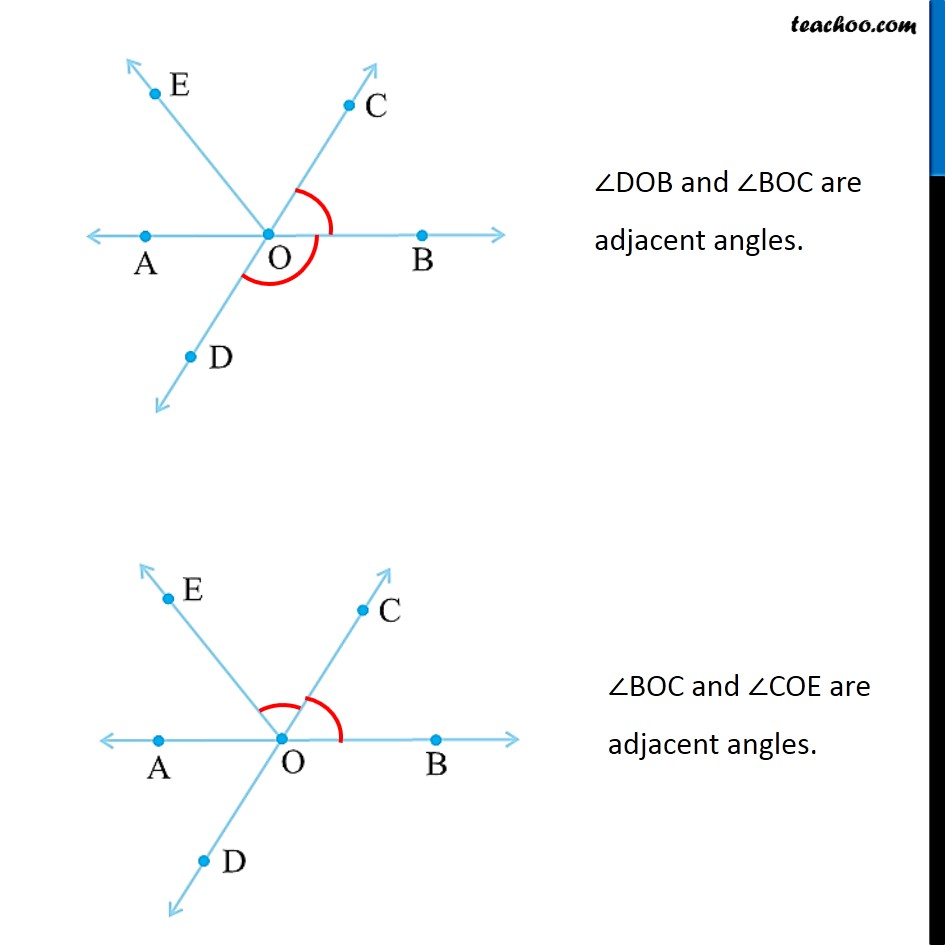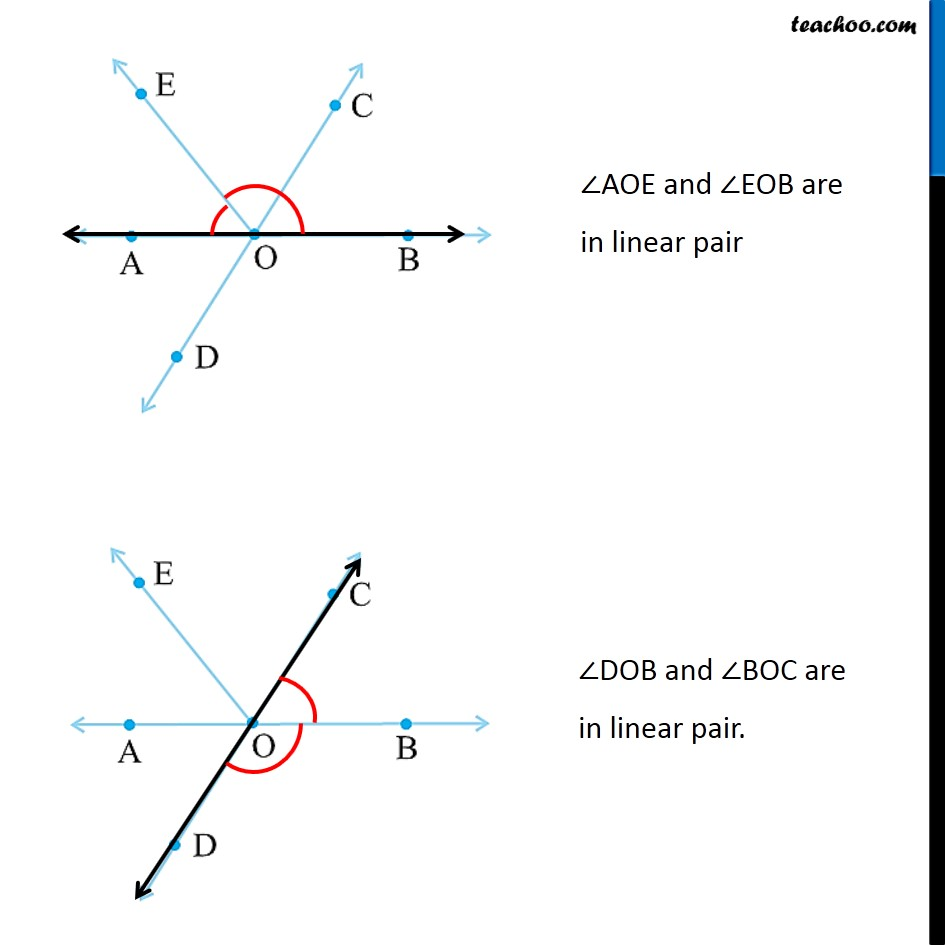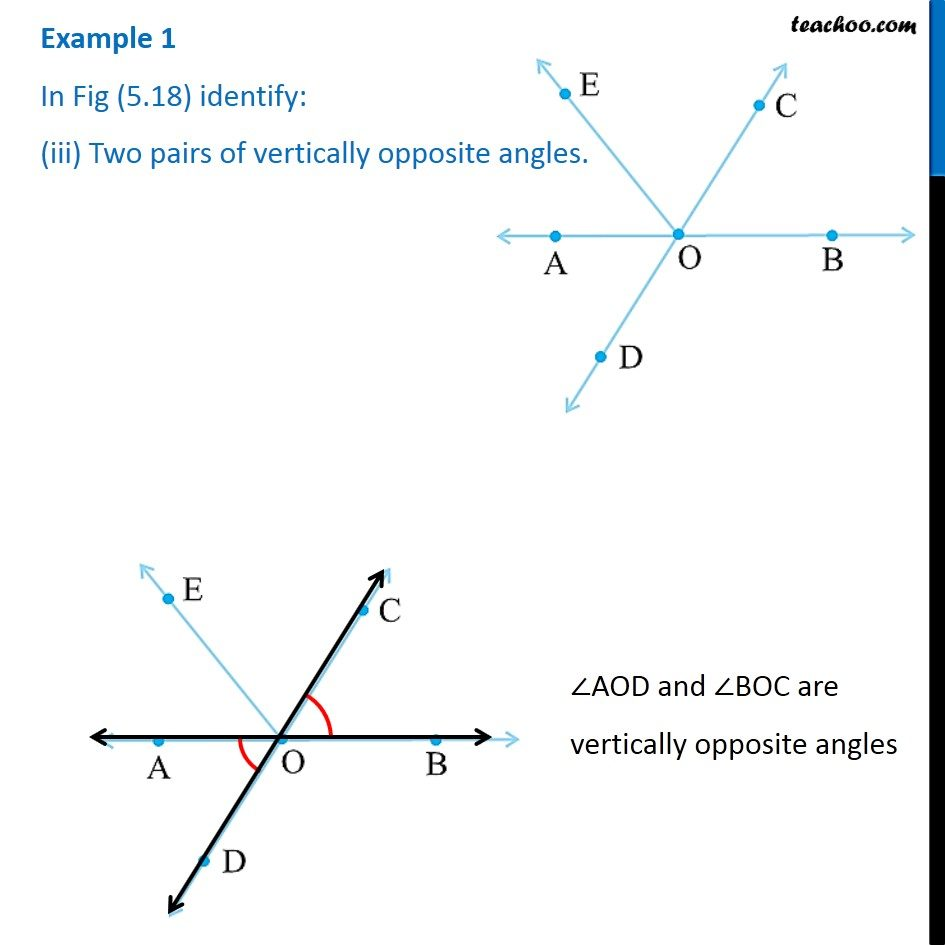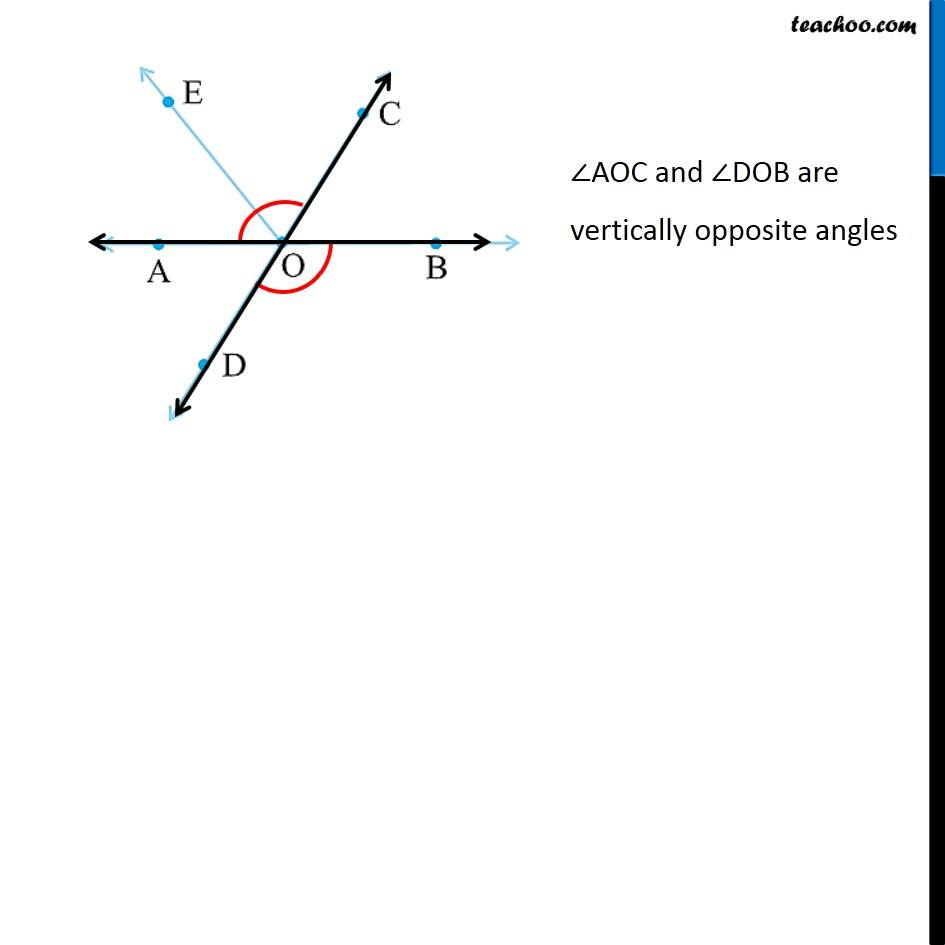1. Chapter 5 Class 7 Lines and Angles
2. Serial order wise

Transcript

Example 1 In Fig (5.18) identify: (i) Five pairs of adjacent angles. Adjacent angles have Common vertex Common side No overlap ∠AOD and ∠DOB are adjacent angles. ∠DOB and ∠BOC are adjacent angles. ∠BOC and ∠COE are adjacent angles. ∠COE and ∠EOA are adjacent angles. ∠EOA and ∠AOD are adjacent angles. ∠AOD and ∠DOB are in linear pair ∠AOE and ∠EOB are in linear pair ∠DOB and ∠BOC are in linear pair. Example 1 In Fig (5.18) identify: (iii) Two pairs of vertically opposite angles. ∠AOD and ∠BOC are vertically opposite angles ∠AOC and ∠DOB are vertically opposite angles

Chapter 5 Class 7 Lines and Angles
Serial order wise